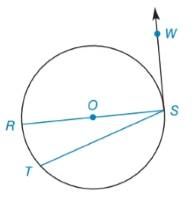Chapter 6.2, Problem 3EElementary Geometry For College St...

7th Edition
Alexander + 2 others
ISBN: 9781337614085

Solutions

Chapter
SectionElementary Geometry For College St...

7th Edition
Alexander + 2 others
ISBN: 9781337614085
Textbook Problem

Given: Circle O with diameter R S ¯ , tangent S W → , chord T S ¯ and m R T ⌢ = 26 ° Find: a ) m ∠ W S R b ) m ∠ R S T c ) m ∠ W S TTo determine

(a)

To find:

mWSR where circle O with diameter RS¯, tangent SW, chord TS¯ and mRT=26°.

Explanation

Theorem:

The radius drawn to a tangent at the point of tangency is perpendicular to the tangent at that point.

Calculation:

To find: mWSR.

To determine

(b)

To find:

mRST when mRT=26°.

To determine

(c)

To find:

mWST.

Still sussing out bartleby?

Check out a sample textbook solution.

See a sample solution

The Solution to Your Study Problems

Bartleby provides explanations to thousands of textbook problems written by our experts, many with advanced degrees!

Get Started

(3, 6)

Applied Calculus for the Managerial, Life, and Social Sciences: A Brief Approach

Find dy/dx by implicit differentiation. 7. x4 + x2y2 + y3 = 5

Single Variable Calculus: Early Transcendentals, Volume I

Solve the equations in Exercises 126. (x21)2(x+2)3(x21)3(x+2)2=0

Finite Mathematics and Applied Calculus (MindTap Course List)

What are the two requirements for a random sample?

Essentials of Statistics for The Behavioral Sciences (MindTap Course List)

Evaluate each expression: 1862446

Elementary Technical Mathematics

True or False: is a convergent series.

Study Guide for Stewart's Multivariable Calculus, 8th